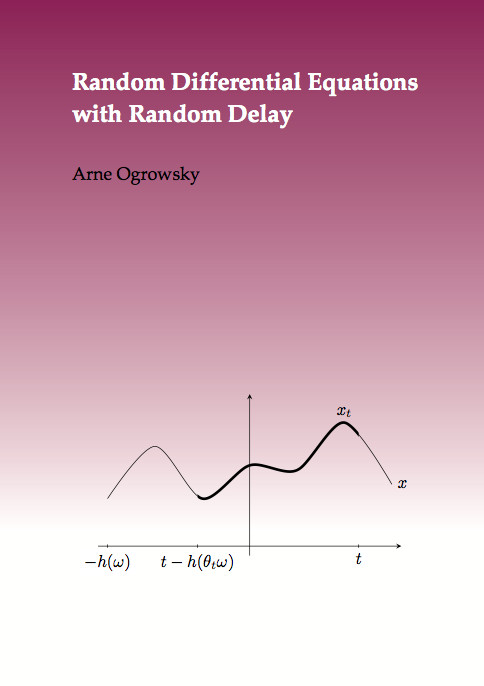Datenbestand vom 19. Juli 2019aktualisiert am 19. Juli 2019

# ISBN 9783843901000Euro 72,00 inkl. 7% MwSt

978-3-8439-0100-0, Reihe Mathematik

Arne Ogrowsky
Random Differential Equations with Random Delay

123 Seiten, Dissertation Universität Paderborn (2011), Hardcover, B5

## Zusammenfassung / Abstract

We deal with a random differential equation of order one. In this differential equation an operator with a discrete spectrum is included as well as a nonlinearity modeling the randomness by a metric dynamical system. In particular, the differential equation is assumed to be a retarded differential equation, that means, a differential equation with a delay term. Its delay is contained in the nonlinearity and is modeled by a shifted mapping from the point of view of functional analysis. The essential point is that the delay is also assumed to be random and unbounded. We show the existence of a random dynamical system that is generated by the solution to this random differential equation with unbounded random delay. Having established this result makes it possible to investigate the system with respect to attractors and manifolds. These concepts describe the dynamical behavior of the system and are therefore of fundamental importance. The existence of those concepts is also established.